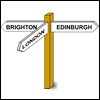# Resources tagged with: Graphing software and graphical calculators

Filter by: Content type:
Age range:
Challenge level:

### There are 10 results

Broad Topics > Physical and Digital Manipulatives > Graphing software and graphical calculators### Quartics

##### Age 16 to 18Challenge Level

Investigate the graphs of y = [1 + (x - t)^2][1 + (x + t^)2] as the parameter t varies.### A Close Match

##### Age 16 to 18Challenge Level

Can you massage the parameters of these curves to make them match as closely as possible?### More Parabolic Patterns

##### Age 14 to 18Challenge Level

The illustration shows the graphs of twelve functions. Three of them have equations y=x^2, x=y^2 and x=-y^2+2. Find the equations of all the other graphs.### Pitchfork

##### Age 16 to 18Challenge Level

Plot the graph of x^y = y^x in the first quadrant and explain its properties.### Parabolic Patterns

##### Age 14 to 18Challenge Level

The illustration shows the graphs of fifteen functions. Two of them have equations y=x^2 and y=-(x-4)^2. Find the equations of all the other graphs.### Exploring Cubic Functions

##### Age 14 to 18Challenge Level

Quadratic graphs are very familiar, but what patterns can you explore with cubics?### Ellipses

##### Age 14 to 18Challenge Level

Here is a pattern for you to experiment with using graph drawing software. Find the equations of the graphs in the pattern.### Parabolas Again

##### Age 14 to 18Challenge Level

Here is a pattern composed of the graphs of 14 parabolas. Can you find their equations?### Climbing

##### Age 16 to 18Challenge Level

Sketch the graphs of y = sin x and y = tan x and some straight lines. Prove some inequalities.### Limiting Probabilities

##### Age 16 to 18Challenge Level

Given probabilities of taking paths in a graph from each node, use matrix multiplication to find the probability of going from one vertex to another in 2 stages, or 3, or 4 or even 100.